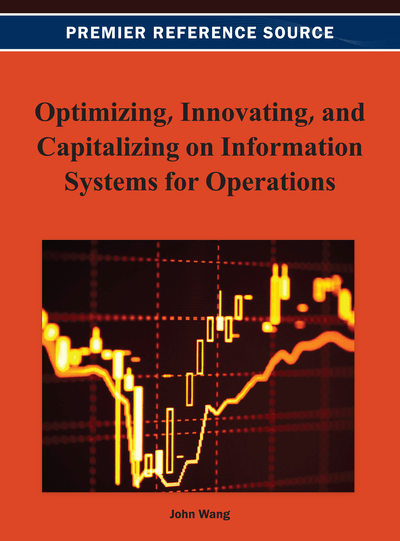# Portfolio Selection Models and Their Discrimination

DOI: 10.4018/978-1-4666-2925-7.ch009
OnDemand:
(Individual Chapters)
Available
\$29.50
No Current Special Offers

## Abstract

The stochastic nature of financial markets is a barrier for successful portfolio management. Besides traditional Markowitz’s model, many other portfolio selection models in Bayesian and Non-Bayesian frameworks have been developed. Starting with the basic Markowitz model, several cardinal models are used to find optimum portfolios with select stock set. Having developed the regression model of the return of each stock with the market return, the unsystematic part of the uncertainty was used to find the optimum portfolio and efficient risk–return frontier within each portfolio selection model. The average stock return as estimated from its historical data and the forecasted stock return were used for maximizing return with quadratic programming formulation in Markowitz model. In the models involving Fuzzy probability and possibility distributions, the future return was estimated using the similarity grade of past returns. In the interval coefficient models, future return was estimated as interval variable. The optimum portfolios of different models were widely divergent and DEA was used to identify the model giving the best portfolio with higher appraisal, both overall and by peers, and least Maverick behavior. Use of Signal to Noise ratio proved equally efficient for model discrimination and yielded identical results.
Chapter Preview
Top

## Introduction

The problem of Portfolio optimization for selecting the best portfolio is an area which received constant attention from leading economist, statistician and mathematicians of the world. The foundation of modern portfolio theory for solving portfolio selection problems was laid by Markowitz (1959, 1987). The theory combined probability and optimization theories to model the behaviour of agents of economic change. The agents were assumed to strike a balance between maximizing the reward (quantified as mean return) and minimizing the risk (quantified as the variance of return from the portfolio) of investment decisions thus focusing on the overall risk-reward characteristics. Mathematical representations of return and risk facilitated risk-return trade-off by formulating and solving the investment decision problem as dual objective (profit maximization and risk minimization) optimization problem. Later Rambaud, Perez, Sanchez Granero, and Trinidad Segovia (2009) studied the Markowitz portfolio selection model with Euclidean vector space concept and presented quantitative and explicit solutions of the optimal portfolio. Baptista (2008) studied the problem as total income variance (TIV) minimization problem from investor’s point of view and error variance (TEV) minimization problem from portfolio manager’s point of view.